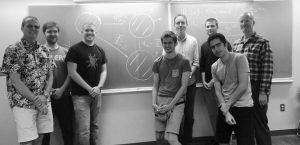# Florida analysis

## Analysis at UFThe analysis group at UF is involved in contemporary research in a broad range of mathematics with important inter and intra-discipline connections. Below is a brief synopsis of the activities. For more information, follow the links to the left to visit individual faculty and the analysis seminar homepages.

#### Complex Analysis and Number Theory

Complex analysis and its applications to special functions including Jacobi elliptic functions, differential equations and number theory.

#### Measure Theory, Commutative and Noncommutative

Infinite dimensional vector-valued integration and stochastic analysis, including stochastic integration which is the basis for much of financial mathematics. One setting for noncommutative measure theory is within a weak-star closed subalgebra of a C-star algebra, while another setting is provided by a (normal) state on a W-star algebra.

#### Partial Differential Equations and Image Processing

Harmonic maps on manifolds; theories and methods of non-linear PDEs. The application of PDEs to image processing, particularly medical such as fMRI. On campus collaborating units include the Brain Institute, the medical school, and computer science. Fully nonlinear PDEs, systems of elliptic and parabolic PDEs related to Algebraic Geometry and prescribed curvature type of equations. Naiver-stokes equations, Nonlinear problems in fluid dynamics and kinetic theory.

#### Sympletic Geometry

Representations of the Weyl and Clifford algebras. Symplectic geometry has its origins in the Hamiltonian formulation of classical mechanics. A symplectic manifold is a differentiable manifolds equipped with a closed, nondegenerate 2-form.

#### Operator Theory and Operator Algebras

Single and several variable operator theory, particularly with connections to analytic functions including interpolation theorems of Pick type which themselves generalize the Schwartz Lemma; composition operators; and index theory. Non-self adjoint operator algebras, and operator systems and spaces. Self-adjoint operator algebras.

#### Noncommutative semi-algebraic geometry

A large class of engineering problem take the form system of dimensionless matrix inequalities; i.e., polynomial inequalities where the variables are matrices and the only restriction on the sizes of the matrices involved is that the formulas make sense. A theory of noncommutative semi-algebraic parallel to the classical commutative theory is rapidly developing to understand matrix inequalities. That there are very few convex noncommutative semi-algebraic sets is one of the most mathematically striking and practically important differences that has emerged to date.

#### Probability, Stochastic Analysis, and Financial Mathematics

Martingales, Probabilistic potential theory. Stochastic integration and financial mathematics. Applications of probability to image processing. High-dimensional probability, concentration of measure, information theory.

Faculty

Students